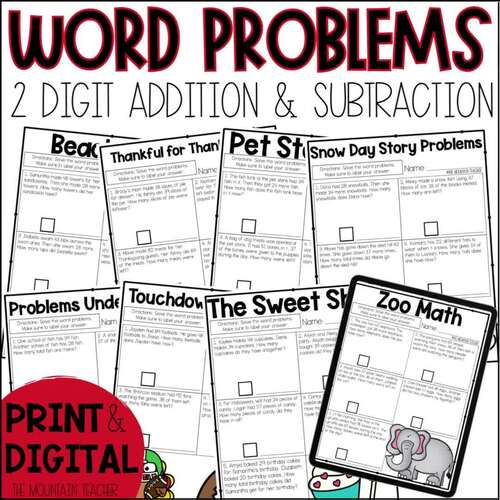Rated 4.79 out of 5, based on 56 reviews
56 Ratings;
1st - 3rd
Subjects
Resource Type
Standards
Formats Included
• PDF
•Google Apps™
Pages
36+
Report this resource to TPTThe Teacher-Author indicated this resource includes assets from Google Workspace (e.g. docs, slides, etc.).
Easel Activity Included
This resource includes a ready-to-use interactive activity students can complete on any device.  Easel by TPT is free to use! Learn more.

#### What educators are saying

This resource made it so easy to have my kids practice word problems. Easy to use, easy for me to check for understanding. Whole group, small group and independent practice - worked well for all.
The themes were fun for the students and the problems were written well. Good variety of problem types.
##### Also included in
1. Planning your 2nd grade math lessons for the year is EASY with this YEAR LONG bundle that includes 40 weeks of 2nd grade math worksheets, activities (printable and digital), homework, pacing guides and assessments for every common core state standard. What's Included With the 2nd Grade Math Workshee
Price \$69.99Original Price \$73.92Save \$3.93

### Description

Need some helpful addition and subtraction word problems worksheets and anchor charts for your 1st, 2nd or 3rd grade students? This 3 week unit is filled with printable and digital addition and subtraction word problems worksheets, assessments and hands-on activities covering taking apart problems, adding to, taking from, putting together, comparing, and more.

What's Included in the Addition and Subtraction Word Problems Worksheets:

• 13 Daily Word Problems Worksheets (Available in a Digital Workbook)
• Pre-Test & Post Test (Printable and Digital)
• 3 Week Teaching Guide
• 3 Days of Homework (Printable and Digital)
• Types of Word Problems Anchor Chart Idea

Formats of Addition and Subtraction Word Problems Worksheets:

• Printable Black and White Worksheets
• Printable Color Worksheets
• Easel Workbook

These Adding and Subtracting Word Problem Worksheets Cover the Following Standards:

• 2.OA.A.1: Use addition and subtraction within 100 to solve one- and two- step word problems involving situations of adding to, taking from, putting together, taking apart and comparing, with unknowns in all positions, e.g., by using drawings and equations with a symbol for the unknown number to represent the problem.
• 2.NBT.B.5: Fluently add and subtract within 100 using strategies based on place value, properties of operations, and/or the relationship between addition and subtraction.
• 2.NBT.B.7: Add and subtract within 1000, using concrete models or drawings and strategies based on place value, properties of operations, and/or the relationship between addition and subtraction; relate the strategy to a written method. Understand that in adding or subtracting three-digit numbers, one adds or subtracts hundreds and hundreds, tens and tens, ones and ones; and sometimes it is necessary to compose or decompose tens or hundreds.

Check Out My Other Second Grade Math Worksheet Packs:

OR check out my Second Grade Let's Do Math Curriculum, including DAILY warm ups, turn and talks, mini lessons, weekly quizzes, unit assessments, benchmark tests, daily worksheets and so much more.

You can also connect with me for first access to my resources, giveaways and FREEBIES via: Email | Website | Instagram | Facebook | Teachers Pay Teachers

Total Pages
36+
Included
Teaching Duration
3 Weeks
Report this resource to TPT
Reported resources will be reviewed by our team. Report this resource to let us know if this resource violates TPT’s content guidelines.

### Standards

to see state-specific standards (only available in the US).
Add within 100, including adding a two-digit number and a one-digit number, and adding a two-digit number and a multiple of 10, using concrete models or drawings and strategies based on place value, properties of operations, and/or the relationship between addition and subtraction; relate the strategy to a written method and explain the reasoning used. Understand that in adding two-digit numbers, one adds tens and tens, ones and ones; and sometimes it is necessary to compose a ten.
Fluently add and subtract within 100 using strategies based on place value, properties of operations, and/or the relationship between addition and subtraction.
Add and subtract within 1000, using concrete models or drawings and strategies based on place value, properties of operations, and/or the relationship between addition and subtraction; relate the strategy to a written method. Understand that in adding or subtracting three-digit numbers, one adds or subtracts hundreds and hundreds, tens and tens, ones and ones; and sometimes it is necessary to compose or decompose tens or hundreds.
Use addition and subtraction within 100 to solve one- and two-step word problems involving situations of adding to, taking from, putting together, taking apart, and comparing, with unknowns in all positions, e.g., by using drawings and equations with a symbol for the unknown number to represent the problem.
Solve two-step word problems using the four operations. Represent these problems using equations with a letter standing for the unknown quantity. Assess the reasonableness of answers using mental computation and estimation strategies including rounding.